﻿ 管路敷设橡胶层对圆柱壳结构振动与声辐射的影响
 舰船科学技术2017, Vol. 39Issue (2): 70-74PDF

Effect on cylindrical shell structural vibration and acoustic radiation due to rubbers laying on pipeline
LIU Fan, ZHOU Qi-dou, LV Xiao-jun
Naval University of Engineering, Wuhan 430033, China
Abstract: A method called structural finite element coupled with fluid boundary element was adopted to calculate the underwater vibration and acoustic characters from a cylindrical shell including a pipeline was affected by the pipeline that surrounded by rubbers different in thickness. The effect of rubbers on the cylindrical shell underwater vibration and acoustic radiation was analyzed from vibration displacement and radiation noise of the cylindrical shell. This provides references for designing acoustic configuration of a underwater structure. The calculation showed that the pipeline surrounded by rubbers different in thickness also decreased the cylindrical shell's underwater radiation noise and vibration for this calculate example. And the thicker rubbers are, the more structural vibration and acoustic radiation are reduced.
Key words: cylindrical shell     pipeline     vibration     acoustic field     rubber layer
0 引 言

1 结构有限元耦合流体边界元算法

 $\left[ {{\mathit{\boldsymbol{K}}}_{s}}-{{\omega }^{2}}{{\mathit{\boldsymbol{M}}}_{s}}-i\omega {{\mathit{\boldsymbol{N}}}_{s}} \right]\mathit{\boldsymbol{a}}=\mathit{\boldsymbol{F}}+{{\mathit{\boldsymbol{F}}}_{\rm{out}}},\text{，}$ (1)

 ${{\mathit{\boldsymbol{F}}}_{out}} = \left[ { - \left( { - {\omega ^2}{\mathit{\boldsymbol{ M}}}} \right) - \left( { - {\mathit{\boldsymbol{i}}}\omega {\mathit{\boldsymbol{ N}}}} \right)} \right]{\mathit{\boldsymbol{a}}}\text{，}$ (2)

 $\left[ {{{\mathit{\boldsymbol{ K}}}_s} - {\omega ^2}({{\mathit{\boldsymbol{ M}}}_s} + {\mathit{\boldsymbol{ M}}}) - {\mathit{\boldsymbol{i}}}\omega ({{\mathit{\boldsymbol{ N}}}_s} + {\mathit{\boldsymbol{ N}}})} \right]{\mathit{\boldsymbol{a}}} = {\mathit{\boldsymbol{F}}}\text{，}$ (3)

 $W = \frac{1}{2}Re\{ i\omega \sum\limits_{j = 1}^{{m_e}} {{p_j}\delta _j^*{S_j}} \} \text{，}$ (4)
 $< \overline {{V^2}} > = \frac{{{\omega ^2}\sum\limits_{j = 1}^{{m_e}} {\left| {{\delta _j}} \right|{S_{\rm{j}}}} }}{{\sum\limits_{j = 1}^{{m_e}} {{S_{\rm{j}}}} }} \text{，}$ (5)

 ${L_W} = 10\lg \frac{W}{{{W_{ref}}}}\text{，}$ (6)
 ${L_{\bar V}} = 10\lg \frac{{ < {{\bar V}^2} > }}{{V_{ref}^2}}\text{。}$ (7)

2 几何模型及有限元建模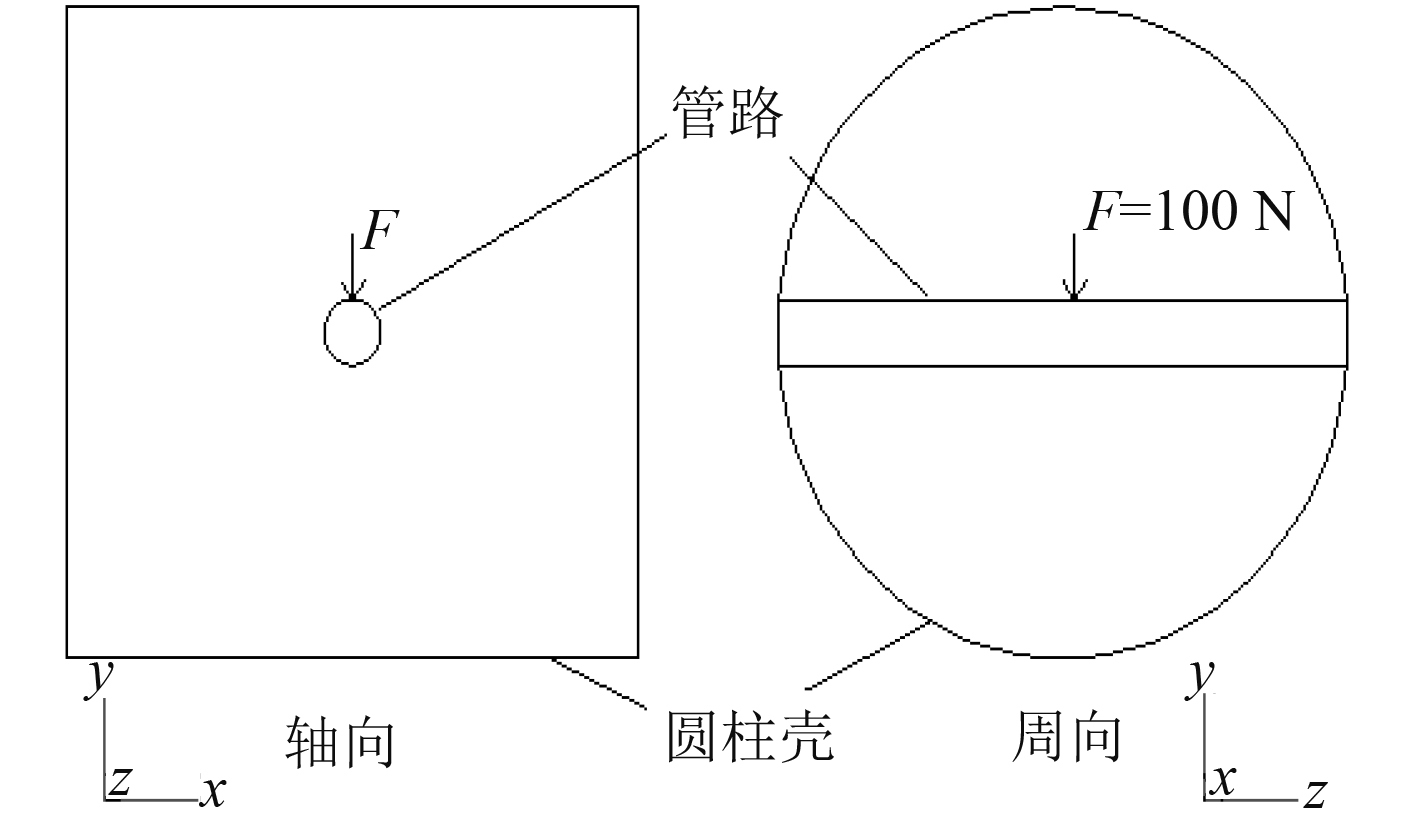图 1 圆柱壳与管路基本结构 Fig. 1 Fundamental structure of the cylindrical shell and pipeline表 1 圆柱壳结构参数 Tab.1 Parameters of the cylindrical shell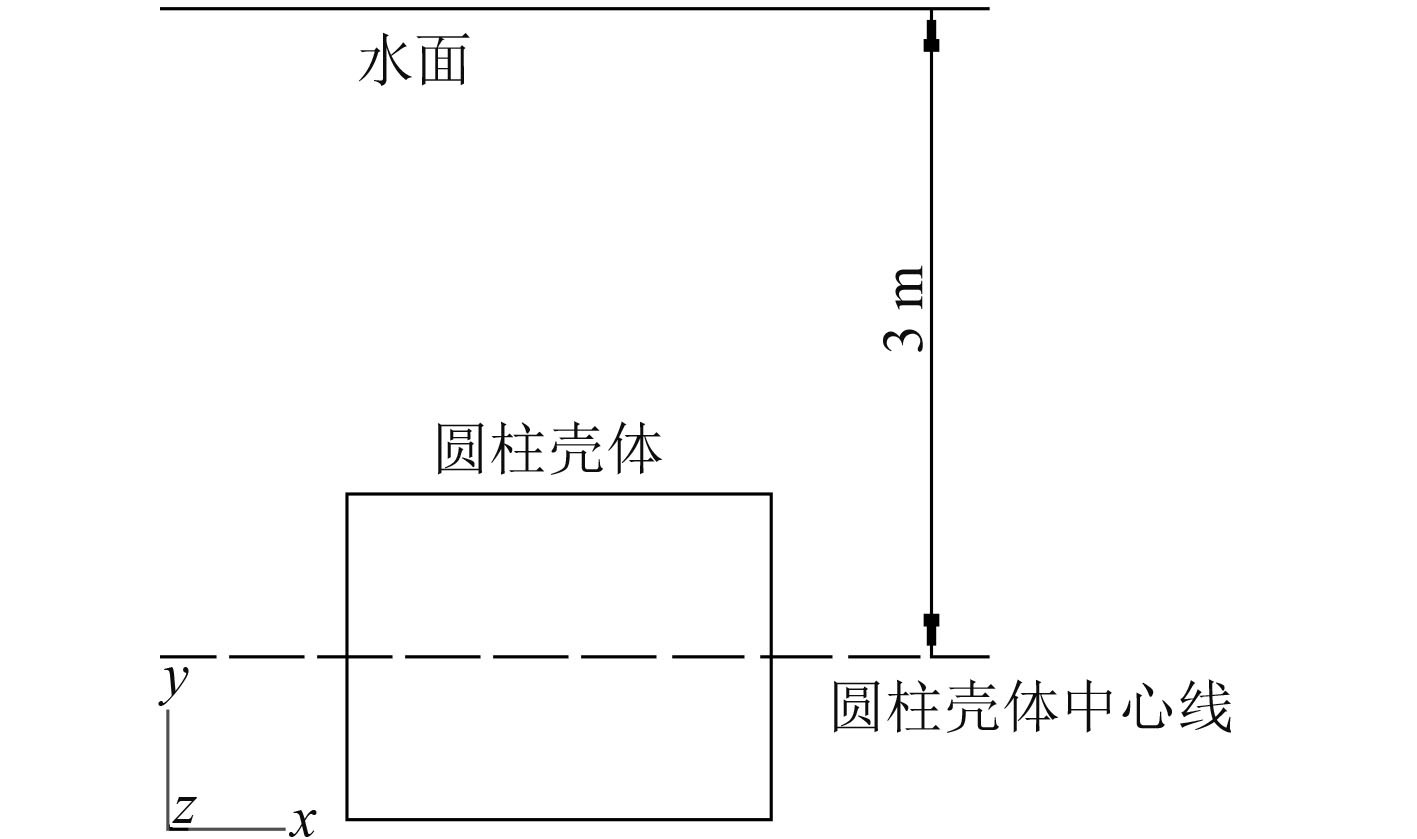图 2 圆柱壳体在水下的位置 Fig. 2 Location of underwater cylindrical shell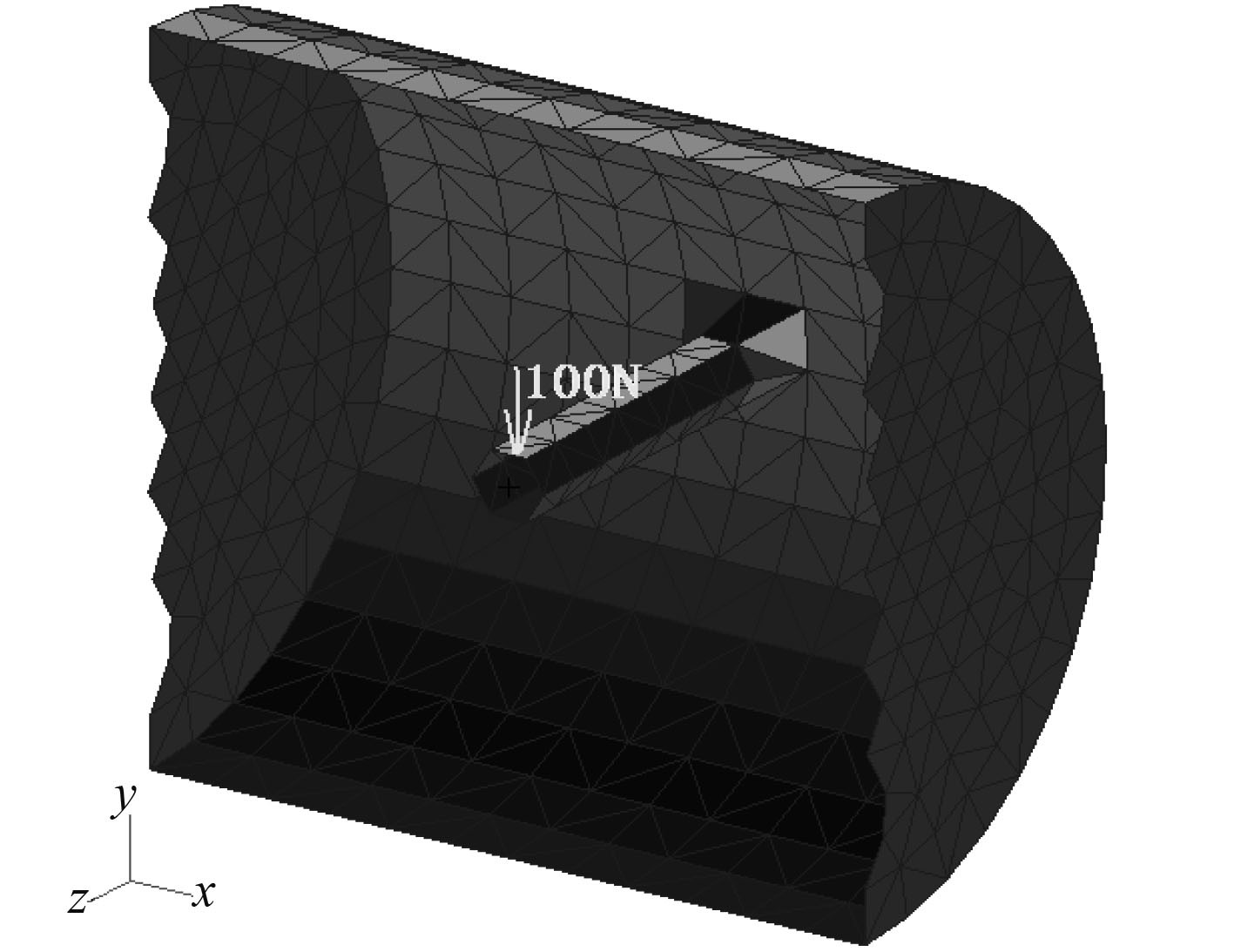图 3 结构有限元模型剖面图 Fig. 3 Structural FE model profile表 2 材料属性 Tab.2 Material property表 3 五种计算模型 Tab.3 Five calculation models
3 数值计算及结果比较分析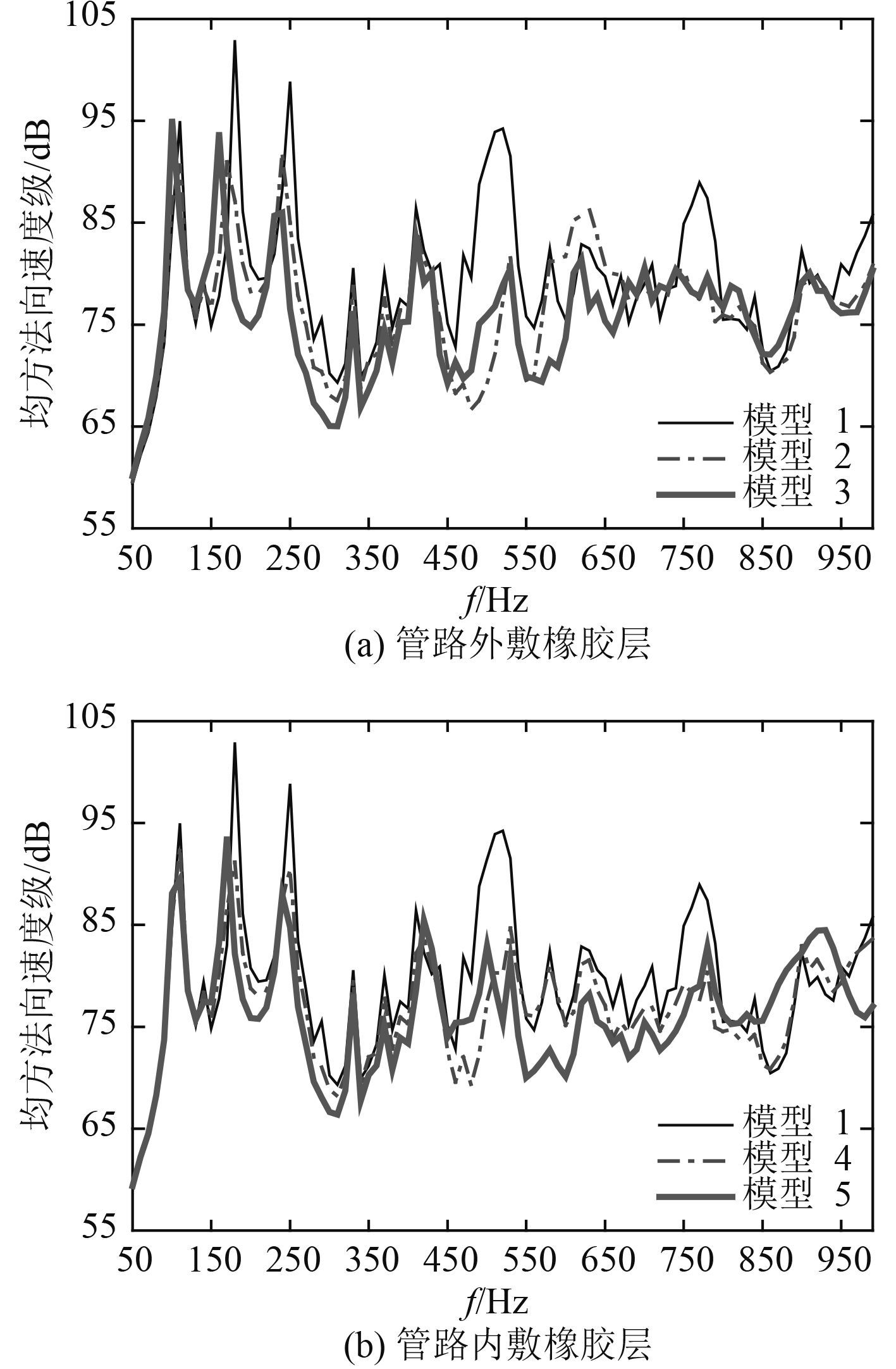图 4 圆柱壳湿表面均方法向速度级频响曲线 Fig. 4 Mean-square normal velocity level frequency response curves of cylindrical shell wetted surface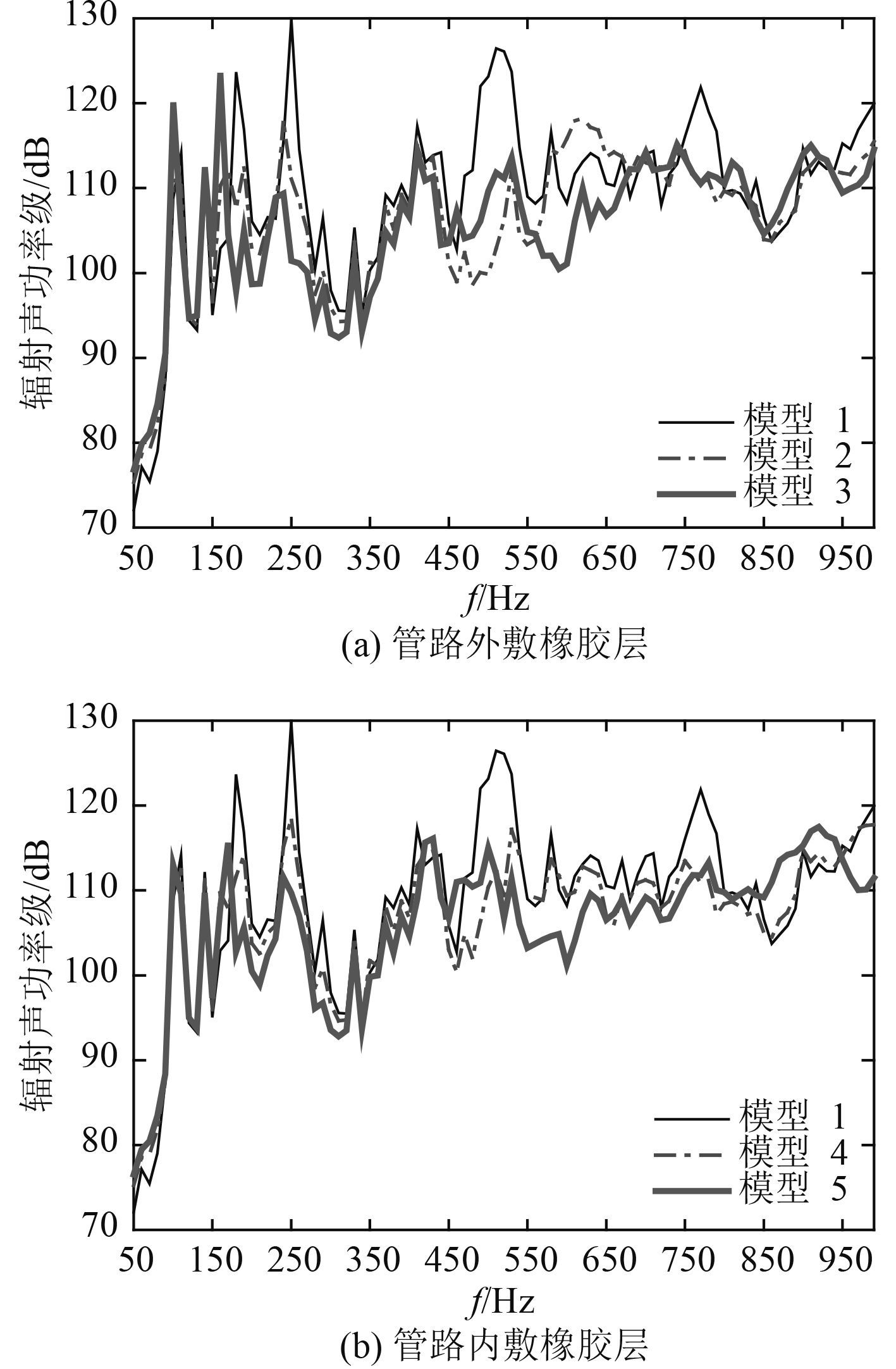图 5 圆柱壳湿表面辐射声功率级频响曲线 Fig. 5 Radiated acoustic power level frequency response curves of cylindrical shell wetted surface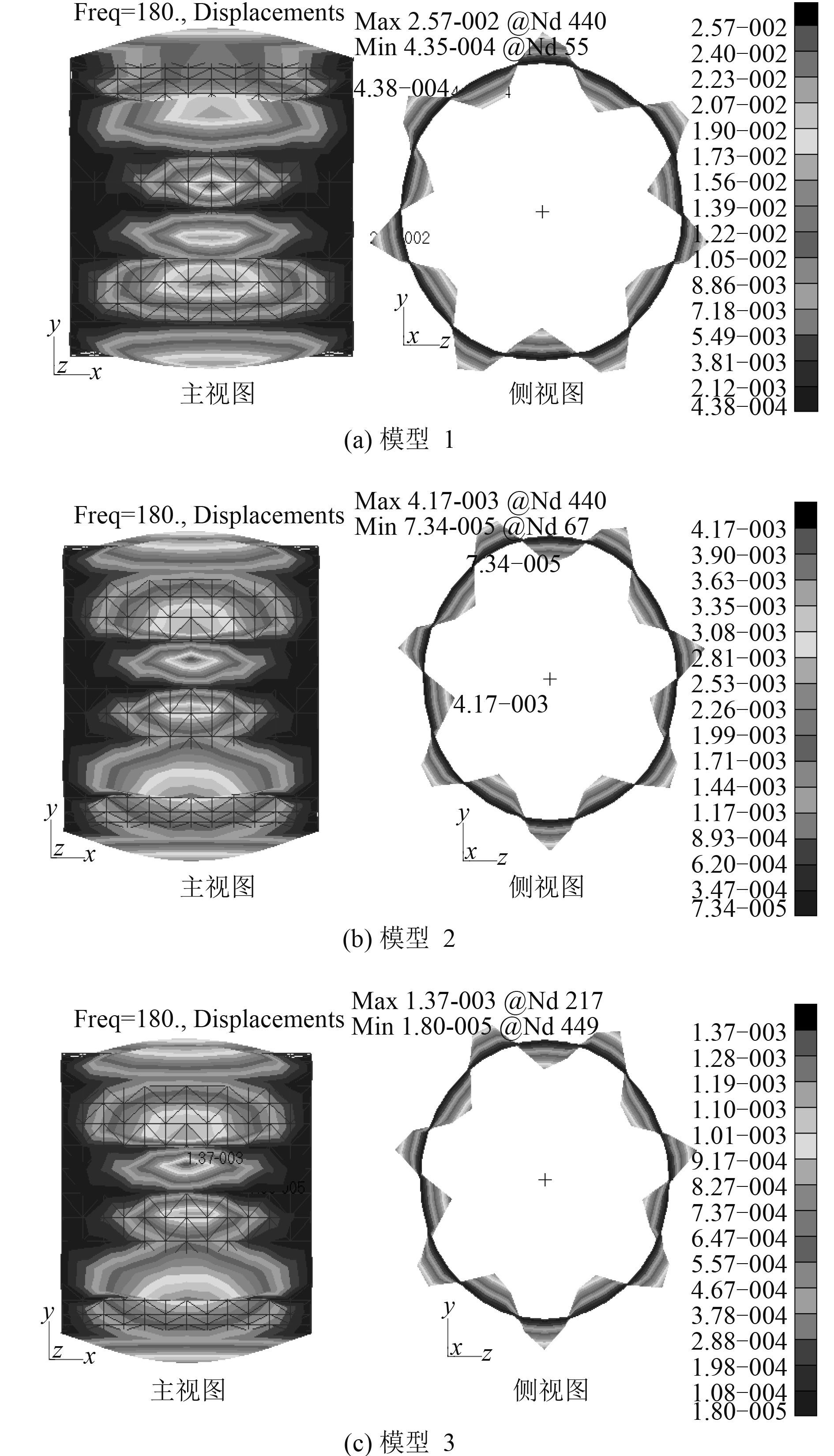图 6 圆柱壳湿表面位移云图 Fig. 6 Displacement coutours of cylindrical shell wetted surface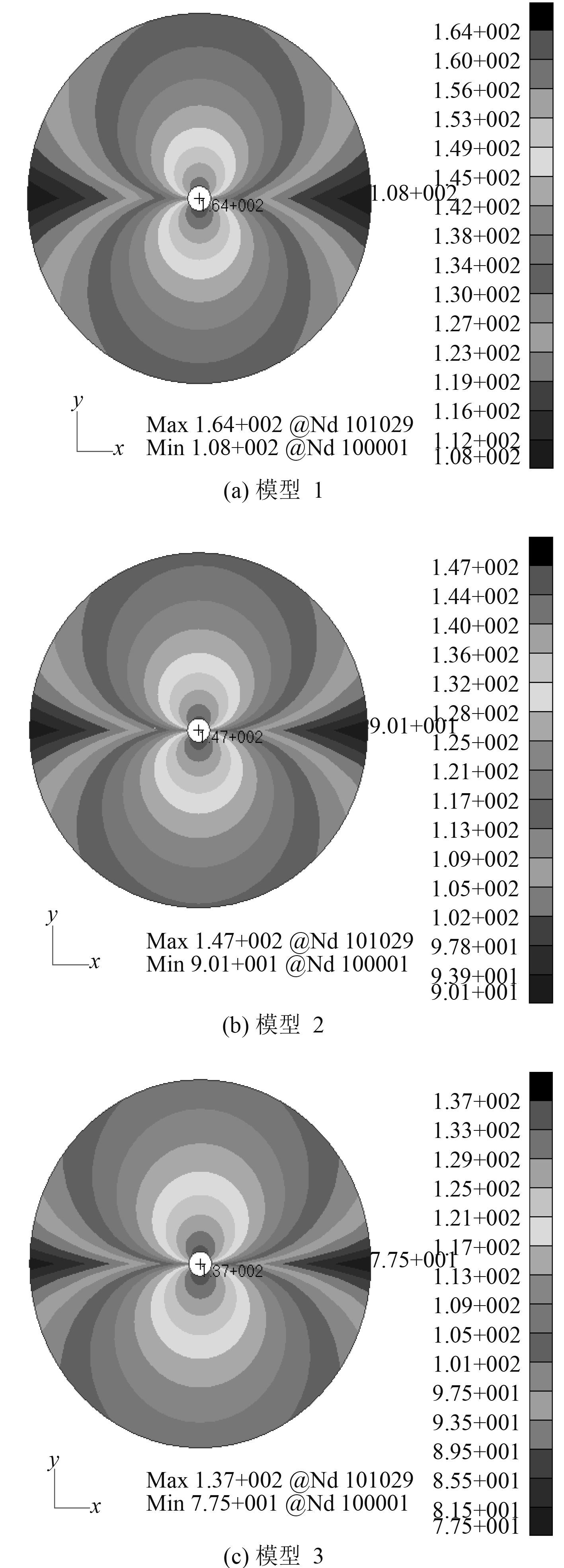图 7 圆柱壳辐射声场声压级云图 Fig. 7 Radiated pressure coutours of acoustic filed out of cylindrical shell

4 结 语

  王仲, 陈长征, 孔祥军. 阻尼对水泵管路隔振系统的影响研究[J]. 重型机械, 2015 (1): 59–62.  王育平, 张丹才, 余永丰. 通海系统管路阻尼处理降噪技术研究[J]. 噪声与振动控制, 2010, 30 (4): 40–43.  梁向东, 付爱华. 非金属阻尼与挠性接管在管路减振中的作用[J]. 振动与噪声控制, 2004 (6): 18–21.  张彩坤, 岳海. 橡胶在水声隐身技术中的应用[J]. 橡胶资源利用, 2007 (3): 3–6.  杜冬菊, 刘爱华, 黄佳典. 阻尼减振在潜艇管路上的应用研究[J]. 中国修船, 2004 (1): 14–16.  刘帆, 周其斗, 纪刚, 等. 纵桁分布方式对结构振动与声辐射的影响[J]. 兵器装备工程学报, 2006 (1): 142–146.  ZHOU Q, ZHANG W, JOSEPH P F. A new method for determining acoustic added mass and damping coefficients of fluid-structure interaction, in:Y. S. Wu, et al (Eds), The Eighth International Symposium on Practical Design of Ships and Other Floating Structures, Elsevier, Amsterdam, 2001, pp. 1185-1195.  ZHOU Q, JOSEPH P F. A numerical method for the calculation of dynamic response and acoustic radiation from an underwater structure[J]. Journal of Sound and Vibration, 2005, 283 (3/5): 853–873.  纪刚, 张纬康, 周其斗. 静水压力作用的水下结构振动及声辐射[J]. 中国造船, 2006, 47 (3): 37–44.